# How to convert decimal Seconds into time format in Excel

In this article, we will learn about how to convert seconds (in decimal) to Seconds (in Time format).

Excel Stores Time in General number format. So to convert the number in decimals to time format. We need to do this process using mathematical calculation.

Here we need to know that excel To convert seconds in decimal format to a proper Excel time, divide by 86400.

1 second = 1/86400 = 0.0000115741 = 0:01

Here we have some examples for explaination.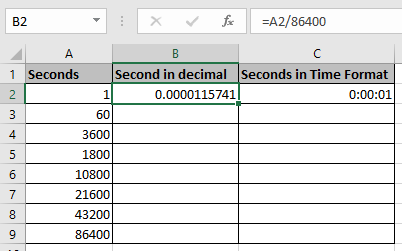First, convert all the seconds to decimals and then convert them to Time format.

Use the formula

=A3/86400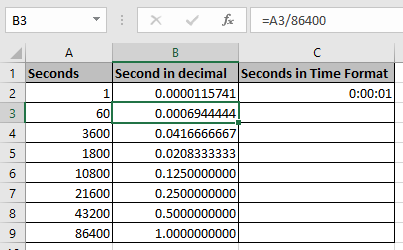As you can see, we have the seconds in decimal format for the time being.

Now I will pick the values and paste them in the Column and change their cell format to Time format.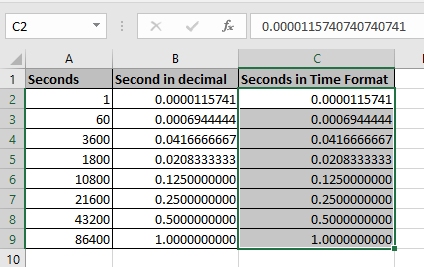Select the cells needed to change the format of the cells.

Then Use Ctrl + 1 to open the format cell dialog box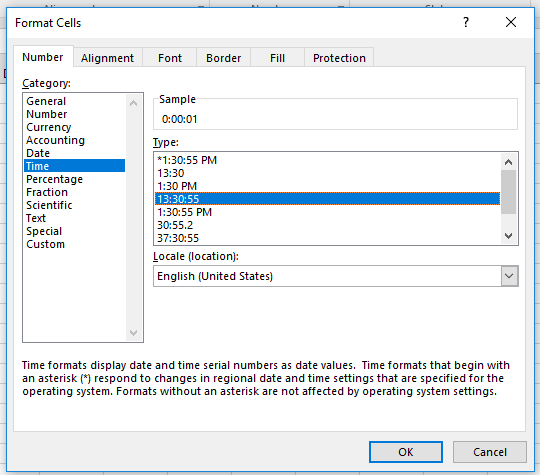Select the Time from category and then select the time format you wish. In this case we are considering 00:00:00(hh:mm:ss).

Click Ok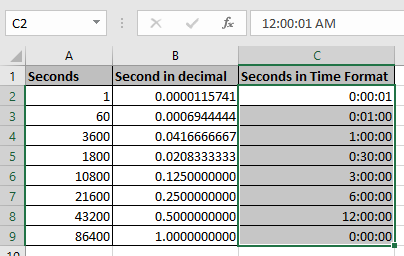As you can see the time format for the seconds(in decimals).
You must be noticing why there is 0:00:00 in C9 cell because 86400 seconds equals one day. So time again starts from the 0:00:00. But the date will be increased by one if considered.

Hope you understood how to convert time in seconds to time in Time format. Explore more articles on Excel Date & Time. Please feel free to state your queries in the comment box below. We will assist you.

Related Articles:

How to use the IF and Conditional formatting in Excel

How to Conditional Formatting with formula in Excel

How to Use the Custom Format Cell in Excel

How to perform Conditional Formatting using VBA in Microsoft Excel

Popular Articles:

50 Excel Shortcuts to Increase Your Productivity

How to use the VLOOKUP Function in Excel

How to use the COUNTIF function in Excel

How to use the SUMIF Function in Excel

Terms and Conditions of use

The applications/code on this site are distributed as is and without warranties or liability. In no event shall the owner of the copyrights, or the authors of the applications/code be liable for any loss of profit, any problems or any damage resulting from the use or evaluation of the applications/code.